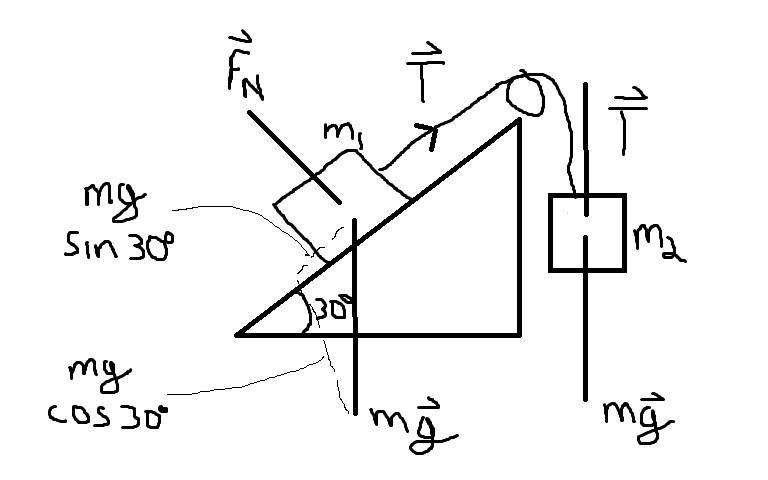# Frictionles force problem with hanging mass

Shapeup

## Homework Statement

A block if mass M1=3.70 Kg on a frictionless plane inclined at 30 degrees is connected by a cord over a massless, frictionless pulley to a second block of mass M2= 2.30Kg. What is the magnitude of acceleration of both blocks?

F=ma

## The Attempt at a SolutionFnormal-M1gsin(30)=0
T=M1a
T-M2g=May

Not really sure where to go from here since I have 2 variables T and a.
T-

Homework Helper
Gold Member

## Homework Statement

A block if mass M1=3.70 Kg on a frictionless plane inclined at 30 degrees is connected by a cord over a massless, frictionless pulley to a second block of mass M2= 2.30Kg. What is the magnitude of acceleration of both blocks?

F=ma

## The Attempt at a Solution

Fnormal-M1gsin(30)=0
your geometry/trig is off, it's not the sin function here...
T=M1a
you are missing a force component in this equation
T-M2g=May
..or is it M2g -T = M2ay?? Or does it matter?
Not really sure where to go from here since I have 2 variables T and a.
T-
2 equations, 2 unknowns..are the accelerations of the masses the same?

Homework Helper
Since M2*g > M1*g*sinθ, M2 moves in the downward direction.
Now write down expression for net force on M1 and M2.